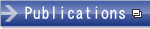HOME > Faculty and Staff > Faculty

## Faculty and Staff

﻿

### Yoshihiro UedaDegree
Ph. D of Mathematical Science (Kyushu University, 2008)
Educational Contents
Undergraduate Course: Linear Algebra 1, 2, Applied Mathematics 3
Research Interests
My research theme is the mathematical analysis of nonlinear partial differential equations describing various natural phenomenon. Especially, I am mainly interested in equations appearing in the fluid mechanics. My purpose of the research is to analyze properties of solutions of mathematical problems.
Message for Students
Mathematics is a base of a lot of studies (engineering, economics, etc.). Thus, we can find the utility of mathematics at various scenes in the world. I hope to study the mathematics research from the viewpoint of application with you.
Research Areas
Nonlinear analysis, Partial differential equations
Affiliations
The Mathematical Society of JapanPageTop